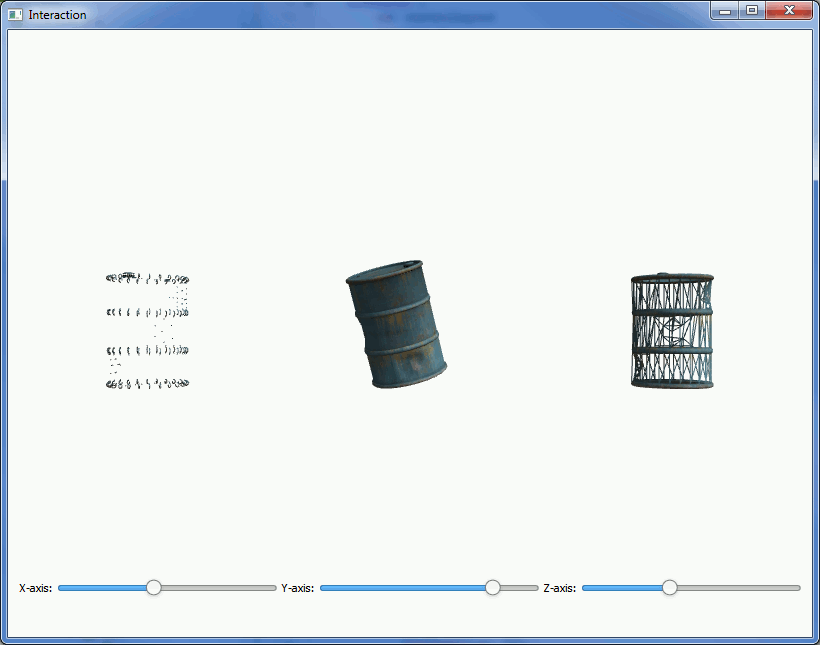# Interaction Example

Interact with an object in canvas using Qt Quick Controls.

The Interaction Example concentrates on the interaction between Qt Quick Controls and the objects displayed in Canvas3D. This also demonstrates drawing the same object in three different ways.## The Controls

First, we need to import Qt Quick Controls and Layouts:

```import QtQuick.Controls 1.0
import QtQuick.Layouts 1.0```

Then, we add a RowLayout to the main component to easily add the controls side-by-side:

```RowLayout {
id: controlLayout
spacing: 5
x: 12
y: parent.height - 100
width: parent.width - (2 * x)
height: 100
visible: true```

And then we add three sliders to the layout. Here's the one for controlling x-axis rotation:

```Slider {
id: xSlider
Layout.alignment: Qt.AlignLeft
Layout.fillWidth: true
minimumValue: 0;
maximumValue: 360;
onValueChanged: canvas3d.xRotSlider = value;
}```

## Interaction

First we need to define some properties in the Canvas3D for the rotations:

```property double xRotSlider: 0
property double yRotSlider: 0
property double zRotSlider: 0```

The `onValueChanged` signal handlers of the Slider components are connected to the above properties. Here's the connection in x-rotation slider as an example:

`onValueChanged: canvas3d.xRotSlider = value;`

Then, on the JavaScript side, we just use the Canvas3D properties directly when setting the modelview matrix rotations:

```mvMatrix = mat4.rotate(mvMatrix, mvMatrix, degToRad(canvas.xRotSlider), [1, 0, 0]);
mvMatrix = mat4.rotate(mvMatrix, mvMatrix, degToRad(canvas.yRotSlider), [0, 1, 0]);
mvMatrix = mat4.rotate(mvMatrix, mvMatrix, degToRad(canvas.zRotSlider), [0, 0, 1]);```

## Three Ways of Drawing

The actual drawing is no different from any situation where the same object is drawn multiple times. Only in this case we use a different drawing mode for each:

```gl.drawElements(gl.TRIANGLES, theModel.count, gl.UNSIGNED_SHORT, 0);
...
gl.drawElements(gl.POINTS, theModel.count, gl.UNSIGNED_SHORT, 0);
...
gl.drawElements(gl.LINES, theModel.count, gl.UNSIGNED_SHORT, 0);```

Files:

Images: